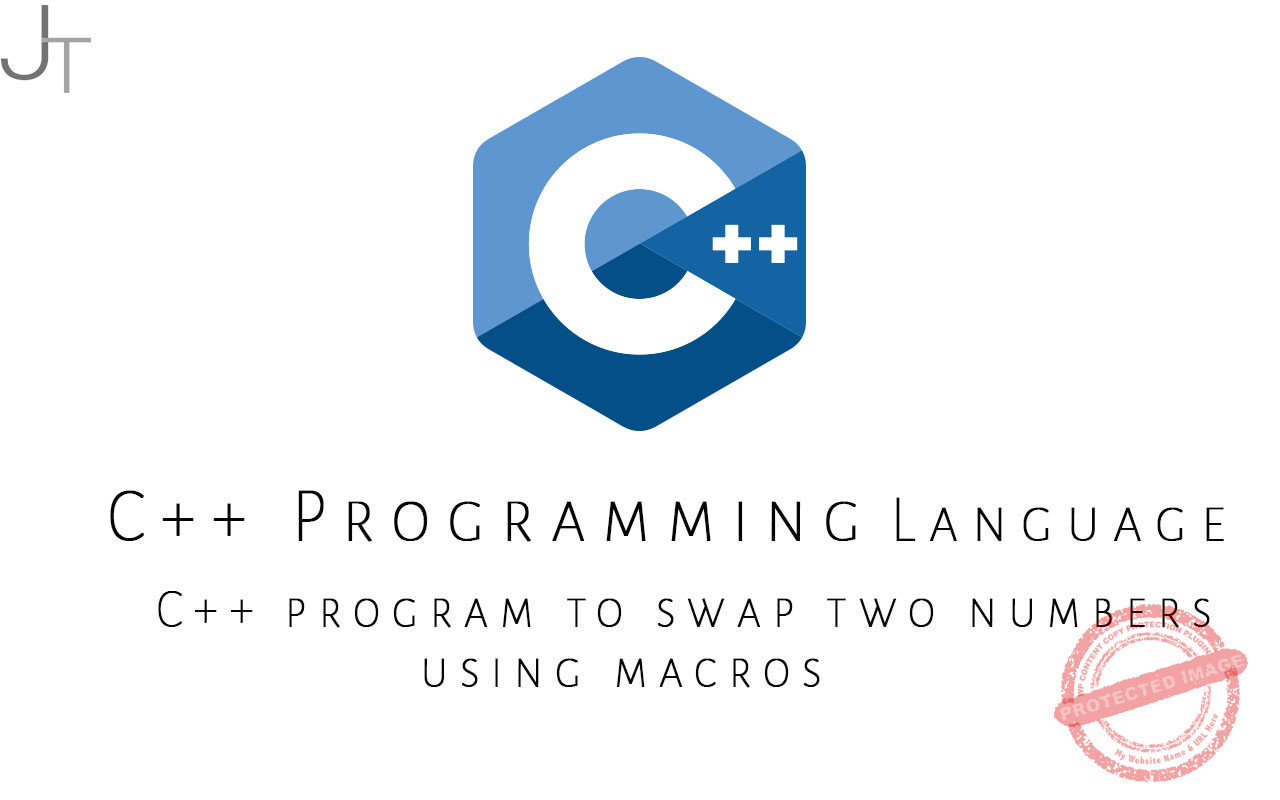# C++ program to swap two numbers using macros``````#include<iostream.h>
#include<conio.h>

#define SWAP(a,b) {int temp; temp=a; a=b; b=temp;}

void main()
{
clrscr();
int x,y;
cout<<“Enter two numbers:”;
cin>>x>>y;

cout<<“x=”<<x<<” y=”<<y;
SWAP(x,y);
cout<<“nx=”<<x<<” y=”<<y;
getch();
}``````

Output

### Recent Posts

error: Alert: Content is protected!!